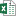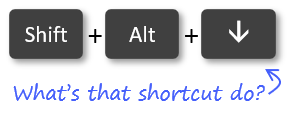# How to Use the Subtotal Feature and the SUBTOTAL Function in Excel

Bottom Line: Learn how the SUBTOTAL function works and how to automatically create subtotal rows in a data set with the Subtotal feature of Excel.

Skill Level: Intermediate

## Video Tutorial

Here is a copy of the file that I use in the video.Subtotal Feature And SUBTOTAL Function.xlsx (24.9 KB)

## The SUBTOTAL Function

In Excel, there is a SUBTOTAL function and a Subtotal feature, and they are different things. Both are useful and I will explain the difference. Let's start with the SUBTOTAL Function.

One great use for the SUBTOTAL function is in the Total Row of an Excel Table. I talk about that in this post: The SUBTOTAL Function for Excel Tables Total Row Explained. But for today's post, I'd like to take a look at how the SUBTOTAL function is used in data sets that have more of an outline setup. These data sets usually have rows grouped together with subtotals and a grand total at the bottom. Here's an example:

The SUBTOTAL Function has two arguments.

1. The first argument identifies what type of calculation you are looking for. This could be an average, a sum, a count, a standard deviation, etc. Each of these types of calculation is assigned a number by Excel.  1 through 11 calculate based on all of the cells in a given data range, while 101 through 111 calculate only the cells that are visible in a data range. For our outline format, the best choice is 9, which is the designation for a sum of the all cells in a specified range, whether visible or not.
1. The second argument is the reference. This is simply the data range that you want the subtotal to calculate from. You can include more than one reference if you want the subtotal to include data from non-contiguous ranges.

## Some Benefits of the SUBTOTAL Function

### The SUBTOTAL Function Doesn't Double Count

One of the nice features about the SUBTOTAL Function is that it recognizes other subtotals within the referenced range and it excludes them from its calculation. This is good because you won't be double-counting the data. If you used the SUM function to add all of the numbers in a range that includes a subtotal, your result would be incorrect.

### The SUBTOTAL Function Saves Time

If you are used to using the SUM function often, you might be tempted to write a SUM formula that simply adds up all of the subtotals. While that may be an easy alternative if you have just a few subtotals in your data set, anything more than that will cost you more time than just using SUBTOTAL. This is especially true if you have dozens or hundreds of subtotal entries.

The SUBTOTAL function automatically adds up the correct data and also has the advantage of being easily changed if you'd like to see another calculation such as an average or a count, while still avoiding duplication from other subtotals in the data range.

## The Subtotal Feature

Separate from the SUBTOTAL function is the Subtotal feature in Excel. This feature is easy to use and is perfect for data that has similar entries or grouped items.

### Prepare the data

The data must be sorted for the column(s) you want to create groups and subtotals for. The Subtotal feature does NOT do this for you. Thanks to Eliot Powell for pointing this out in the comments of the YouTube video.

### Create the Subtotal Outline Groups

To use the Subtotal feature, place your cursor anywhere in the data set. Then click on Subtotal in the Outline section that's located on the Data tab. The Subtotal button looks like this:

That brings up the Subtotal window with options and choices for customizing your subtotals. These options include:

• Designating where you want the subtotals to appear.
• Replacing existing subtotals.
• Adding page breaks between subtotaled sections.
• Placing subtotals in multiple columns.
• And adding a summary or Grand Total line at the bottom.

Once you click OK, the Subtotal and Grand Total Rows are added. These rows use the SUBTOTAL function in the formulas to calculate the sum.

### Expand and Collapse the Row Groups

You will notice that to the left of the row numbers, there are some markings that indicate the span of the subtotals groups, and also the ability to collapse and expand those sections. Number buttons above that indicate levels of the subtotal groupings so that you can drill down or zoom back out to look at more or less detail.

## Free Training Webinar on the Power Tools

Right now I'm running a free training webinar on all of the Power Tools in Excel. This includes Power Query, Power Pivot, Power BI, pivot tables, macros & VBA, and more.

It's called The Modern Excel Blueprint. During the webinar I explain what these tools are and how they can fit into your workflow.

You will also learn how to become the Excel Hero of your organization, that go-to gal or guy that everyone relies on for Excel help and fun projects.

The webinar is running at multiple days and times. Please click the link below to get registered and save your seat.

## Conclusion

You may be thinking that pivot tables have a lot of the same functionality as these subtotaled outlines, and you would be right. I tend to use pivot tables over outlines like this, but it's still great to know how these features work and what can be done with both the SUBTOTAL function and the Subtotal feature. I hope this post has helped with that.

Let me know what questions or comments you have by leaving a note in the comments section. I'd love to hear from you.

•Enrique says:

Hi Jon,
Is it also possible to add subtotals to an existing pivot table (Without destroying the pivot table)? I am working with an older excel version which only has 1 subtotal in the pivot, but I am in need of subtotals of multiple colums.

• Generic filters
Exact matches onlyLearn 10 great Excel techniques that will wow your boss and make your co-workers say, "how did you do that??"

#### Excel Shortcuts ListLearn over 270 Excel keyboard & mouse shortcuts for Windows & Mac.

Excel Shortcuts List